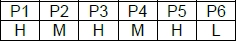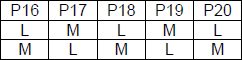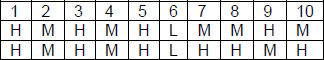# Fuel contamination levels at each of 20 | CAT Logical Reasoning

### Since 2015, the CAT DILR section has become increasingly difficult. In order to tackle the tougher CAT Level DILR questions for the CAT Exam, it is important to understand the basics of CAT. Data Interpretation sets and Logical reasoning puzzles were distinct in DILR. Data Interpretation and Logical Reasoning used to be about computation and understanding charts, graphs, and tables. Within that one hour, you get 5 high-quality puzzles, with some of them being quite challenging. You will solve 30 actual CAT puzzles in CAT 2018 and CAT 2019. The purpose of this page is to help you prepare for that. Take a crack at those puzzles!To obtain a great CAT score, make use of MBAP Free Study material with detailed solutions and video explanations. Check out MBAP free Mock test to take these questions in a test format for free.

You may also find remaining question solution of CAT 2018, slot 1 by searching the question in the search bar.

## CAT 2018 - Slot 1 - Set 6 - Fuel contamination levels at each of 20

##### Fuel contamination levels at each of 20 petrol pumps P1, P2, …, P20 were recorded as either high, medium, or low.1.Contamination levels at three pumps among P1 – P5 were recorded as high.2. P6 was the only pump among P1 – P10 where the contamination level was recorded as low.3. P7 and P8 were the only two consecutively numbered pumps where the same levels of contamination were recorded.4. High contamination levels were not recorded at any of the pumps P16 – P20.5. The number of pumps where high contamination levels were recorded was twice the number of pumps where low contamination levels were recorded.

Q. 1: Which of the following MUST be true?

1. The contamination level at P10 was recorded as high.
2. The contamination level at P13 was recorded as low.
3. The contamination level at P20 was recorded as medium.
4. The contamination level at P12 was recorded as high.

Explanation: According to 1 and 2, we getAlso, from 4, we get 2 cases:From (5)

If total number of low (L) pipes = 3

number of high (H) pipes = 6 number of medium (M) pipes = 11 Also if number of low (L) pipes = 4 number of high (H) pipes = 8 number of medium (M) pipes = 8 P7 and P8 can be HH or MM

Therefore, two cases arise for P1 – P10Combining (1) & (2), we get the following possible cases for P1 – P 20
Case 1:

H M H M H L H H M H M H M H M L M L M L No. (L) = 4
No. (H) = 8

No. (M) = 8

Case 2:

H M H M H L H H M H L M H M H M L M L M No. (L) = 4,
No. (H) = 8,

and No. (M) = 8

Case 3:

H M H M H L H H M H M L H M H M L M L M No. (L) = 4
No. (H) = 8

No. (M) = 8
##### Q. 2: What best can be said about the number of pumps at which the contamination levels were recorded as medium?
1. Exactly 8
2. More than 4
3. At least 8
4. At most 9

Q. 3: If the contamination level at P11 was recorded as low, then which of the following MUST be true?

1. The contamination level at P12 was recorded as high.
2. The contamination level at P14 was recorded as medium.
3. The contamination level at P15 was recorded as medium.
4. The contamination level at P18 was recorded as low.

Q. 4: If contamination level at P15 was recorded as medium, then which of the following MUST be FALSE?

1. Contamination level at P14 was recorded to be higher than that at P15.
2. Contamination levels at P10 and P14 were recorded as the same.
3. Contamination levels at P13 and P17 were recorded as the same.
4. Contamination levels at P11 and P16 were recorded as the same

### Past Year Question Paper & SolutionsCounselling Session
By IIM Mentor

### Free Material Area